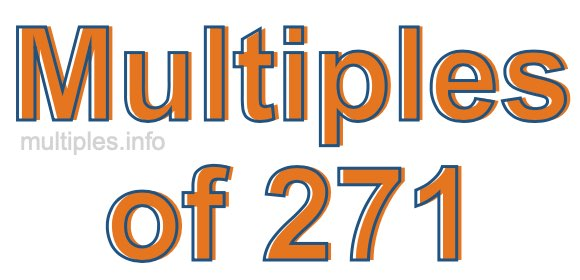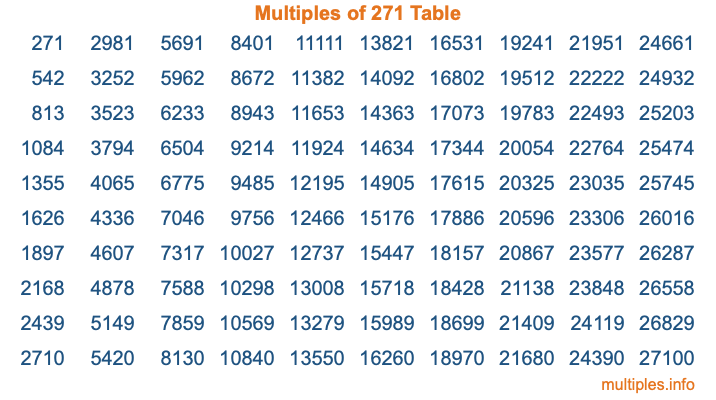Multiples of 271Welcome to the Multiples of 271 page. Here we will first teach you everything you will ever need to know about the multiples of 271, and then give you a study guide summary of everything we taught you to make sure you remember it all. Use this page to look up facts and learn information about the multiples of 271. This page will make you a multiples of two hundred seventy-one expert!

Definition of Multiples of 271
Multiples of 271 are all the numbers that when divided by 271 equal an integer. Each of the multiples of 271 are called a multiple. A multiple of 271 is created by multiplying 271 by an integer.

Therefore, to create a list of multiples of 271, you start with 1 multiplied by 271, then 2 multiplied by 271, then 3 multiplied by 271, and so on for as long as you want. Thus, the list of the first five multiples of 271 is 271, 542, 813, 1084, and 1355. To see a larger list of multiples of 271, see the printable image of Multiples of 271 further down on this page. We also have a category where you can choose any nth multiple of 271.

Multiples of 271 Checker
The Multiples of 271 Checker below checks to see if any number of your choice is a multiple of 271. In other words, it checks to see if there is any number (integer) that when multiplied by 271 will equal your number. To do that, we divide your number by 271. If the the quotient is an integer, then your number is a multiple of 271.

Is  a multiple of 271?

Least Common Multiple of 271 and ...
A Least Common Multiple (LCM) is the lowest multiple that two or more numbers have in common. This is also called the smallest common multiple or lowest common multiple and is useful to know when you are adding our subtracting fractions. Enter one or more numbers below (271 is already entered) to find the LCM.

Check out our LCM Calculator if you need more details about the Least Common Multiple or if you need the LCM for different numbers for adding and subtraction fractions.

nth Multiple of 271
As we stated above, 271 is the first multiple of 271, 542 is the second multiple of 271, 813 is the third multiple of 271, and so on. Enter a number below to find the nth multiple of 271.

th multiple of 271

Multiples of 271 vs Factors of 271
271 is a multiple of 271 and a factor of 271, but that is where the similarities end. All postive multiples of 271 are 271 or greater than 271. All positive factors of 271 are 271 or less than 271.

Below is the beginning list of multiples of 271 and the factors of 271 so you can compare:

Multiples of 271: 271, 542, 813, 1084, 1355, etc.

Factors of 271: 1, 271

As you can see, the multiples of 271 are all the numbers that you can divide by 271 to get a whole number. The factors of 271, on the other hand, are all the whole numbers that you can multiply by another whole number to get 271.

It's also interesting to note that if a number (x) is a factor of 271, then 271 will also be a multiple of that number (x).

Multiples of 271 vs Divisors of 271
The divisors of 271 are all the integers that 271 can be divided by evenly. Below is a list of the divisors of 271.

Divisors of 271: 1, 271

The interesting thing to note here is that if you take any multiple of 271 and divide it by a divisor of 271, you will see that the quotient is an integer.

Multiples of 271 Table
Below is an image of the first 100 multiples of 271 in a table. The table is in chronological order, column by column. The first column has the first ten multiples of 271, the second column has the next ten multiples of 271, and so on.The Multiples of 271 Table is also referred to as the 271 Times Table or Times Table of 271. You are welcome to print out our table for your studies.

Negative Multiples of 271
Although not often discussed or needed in math, it is worth mentioning that you can make a list of negative multiples of 271 by multiplying 271 by -1, then by -2, then by -3, and so on, to get the following list of negative multiples of 271:

-271, -542, -813, -1084, -1355, etc.

Multiples of 271 Summary
Below is a summary of important Multiples of 271 facts that we have discussed on this page. To retain the knowledge on this page, we recommend that you read through the summary and explain to yourself or a study partner why they hold true.

There are an infinite number of multiples of 271.

A multiple of 271 divided by 271 will equal a whole number.

271 divided by a factor of 271 equals a divisor of 271.

The nth multiple of 271 is n times 271.

The largest factor of 271 is equal to the first positive multiple of 271.

271 is a multiple of every factor of 271.

271 is a multiple of 271.

A multiple of 271 divided by a divisor of 271 equals an integer.

271 divided by a divisor of 271 equals a factor of 271.

Any integer times 271 will equal a multiple of 271.

Multiples of a Number
Here you can get the multiples of another number, all with the same attention to detail as we did for multiples of 271 on this page.

Multiples of
Multiples of 272
Did you find our page about multiples of two hundred seventy-one educational? Do you want more knowledge? Check out the multiples of the next number on our list!